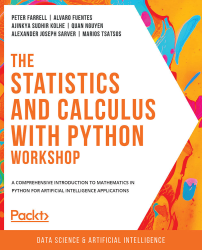•#### The Statistics and Calculus with Python Workshop#### Overview of this book

Are you looking to start developing artificial intelligence applications? Do you need a refresher on key mathematical concepts? Full of engaging practical exercises, The Statistics and Calculus with Python Workshop will show you how to apply your understanding of advanced mathematics in the context of Python. The book begins by giving you a high-level overview of the libraries you'll use while performing statistics with Python. As you progress, you'll perform various mathematical tasks using the Python programming language, such as solving algebraic functions with Python starting with basic functions, and then working through transformations and solving equations. Later chapters in the book will cover statistics and calculus concepts and how to use them to solve problems and gain useful insights. Finally, you'll study differential equations with an emphasis on numerical methods and learn about algorithms that directly calculate values of functions. By the end of this book, you’ll have learned how to apply essential statistics and calculus concepts to develop robust Python applications that solve business challenges.
Preface1. Fundamentals of PythonFree Chapter
2. Python's Main Tools for Statistics3. Python's Statistical Toolbox4. Functions and Algebra with Python5. More Mathematics with Python6. Matrices and Markov Chains with Python7. Doing Basic Statistics with Python8. Foundational Probability Concepts and Their Applications9. Intermediate Statistics with Python10. Foundational Calculus with Python11. More Calculus with Python12. Intermediate Calculus with PythonAppendix# Functions and Algorithms

While functions denote a specific object in Python programming with which we can order and factor our programs, the term algorithm typically refers to the general organization of a sequence of logic to process its given input data. In data science and scientific computing, algorithms are ubiquitous, commonly taking the form of machine learning models that are used to process data and potentially make predictions.

In this section, we will discuss the concept and syntax of Python functions and then tackle some example algorithm-design problems.

## Functions

In its most abstract definition, a function is simply an object that can take in an input and producing an output, according to a given set of instructions. A Python function is of the following form:

```def func_name(param1, param2, ...):
[…]
return […]```

The `def` keyword denotes the start of a Python function. The name of a function can...